## The Pauli Exclusion Principle

### Learning Objectives

By the end of this section, you will be able to:

• Define the composition of an atom along with its electrons, neutrons, and protons.
• Explain the Pauli exclusion principle and its application to the atom.
• Specify the shell and subshell symbols and their positions.
• Define the position of electrons in different shells of an atom.
• State the position of each element in the periodic table according to shell filling.

## Multiple-Electron Atoms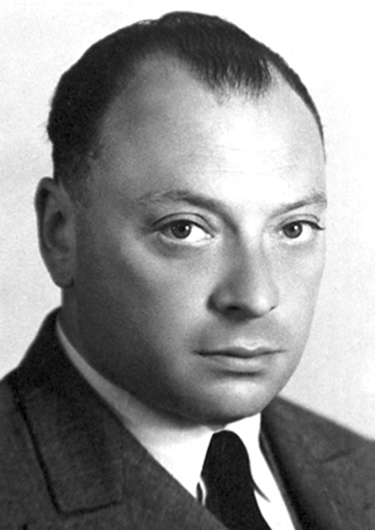Figure 1. The Austrian physicist Wolfgang Pauli (1900–1958) played a major role in the development of quantum mechanics. He proposed the exclusion principle; hypothesized the existence of an important particle, called the neutrino, before it was directly observed; made fundamental contributions to several areas of theoretical physics; and influenced many students who went on to do important work of their own. (credit: Nobel Foundation, via Wikimedia Commons)

All atoms except hydrogen are multiple-electron atoms. The physical and chemical properties of elements are directly related to the number of electrons a neutral atom has. The periodic table of the elements groups elements with similar properties into columns. This systematic organization is related to the number of electrons in a neutral atom, called the atomic number, Z. We shall see in this section that the exclusion principle is key to the underlying explanations, and that it applies far beyond the realm of atomic physics.

In 1925, the Austrian physicist Wolfgang Pauli (see Figure 1) proposed the following rule: No two electrons can have the same set of quantum numbers. That is, no two electrons can be in the same state. This statement is known as the Pauli exclusion principle, because it excludes electrons from being in the same state. The Pauli exclusion principle is extremely powerful and very broadly applicable. It applies to any identical particles with half-integral intrinsic spin—that is, having s = 1/2, 3/2, … Thus no two electrons can have the same set of quantum numbers.

### Pauli Exclusion Principle

No two electrons can have the same set of quantum numbers. That is, no two electrons can be in the same state.

Let us examine how the exclusion principle applies to electrons in atoms. The quantum numbers involved were defined in Quantum Numbers and Rules as nlmls, and ms. Since s is always 1/2 for electrons, it is redundant to list s, and so we omit it and specify the state of an electron by a set of four numbers (nlmlms). For example, the quantum numbers (2, 1, 0, −1/2) completely specify the state of an electron in an atom.

Since no two electrons can have the same set of quantum numbers, there are limits to how many of them can be in the same energy state. Note that n determines the energy state in the absence of a magnetic field. So we first choose n, and then we see how many electrons can be in this energy state or energy level. Consider the n=1 level, for example. The only value l can have is 0 (see Table 1 in Quantum Numbers and Rules for a list of possible values once n is known), and thus ml can only be 0. The spin projection ms can be either +1/2 or −1/2, and so there can be two electrons in the = 1 state. One has quantum numbers (1, 0, 0, +1/2), and the other has (1, 0, 0, −1/2). Figure 2 illustrates that there can be one or two electrons having = 1, but not three.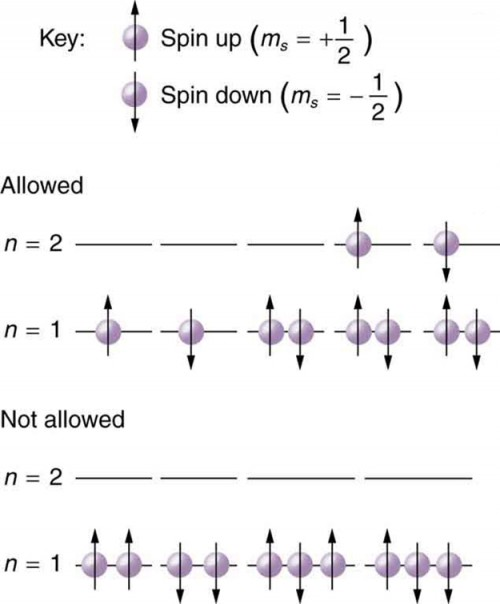Figure 2. The Pauli exclusion principle explains why some configurations of electrons are allowed while others are not. Since electrons cannot have the same set of quantum numbers, a maximum of two can be in the = 1 level, and a third electron must reside in the higher-energy n = 2 level. If there are two electrons in the = 1 level, their spins must be in opposite directions. (More precisely, their spin projections must differ.)

## Shells and Subshells

Because of the Pauli exclusion principle, only hydrogen and helium can have all of their electrons in the = 1 state. Lithium (see the periodic table) has three electrons, and so one must be in the = 2 level. This leads to the concept of shells and shell filling. As we progress up in the number of electrons, we go from hydrogen to helium, lithium, beryllium, boron, and so on, and we see that there are limits to the number of electrons for each value of n. Higher values of the shell n correspond to higher energies, and they can allow more electrons because of the various combinations of lml, and ms that are possible. Each value of the principal quantum number n thus corresponds to an atomic shell into which a limited number of electrons can go. Shells and the number of electrons in them determine the physical and chemical properties of atoms, since it is the outermost electrons that interact most with anything outside the atom.

The probability clouds of electrons with the lowest value of l are closest to the nucleus and, thus, more tightly bound. Thus when shells fill, they start with = 0, progress to = 1, and so on. Each value of l thus corresponds to a subshell.

The table given below lists symbols traditionally used to denote shells and subshells.

Table 1. Shell and Subshell Symbols
Shell Subshell
n l Symbol
1 0 s
2 1 p
3 2 d
4 3 f
5 4 g
5 h
6 i

To denote shells and subshells, we write nl with a number for n and a letter for l. For example, an electron in the n=1 state must have = 0, and it is denoted as a 1s electron. Two electrons in the = 1 state is denoted as 1s2. Another example is an electron in the = 2 state with = 1, written as 2p. The case of three electrons with these quantum numbers is written 2p3. This notation, called spectroscopic notation, is generalized as shown in Figure 3.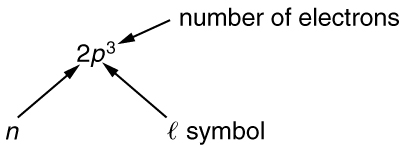Figure 3. Counting the number of possible combinations of quantum numbers allowed by the exclusion principle, we can determine how many electrons it takes to fill each subshell and shell.

### Example 1. How Many Electrons Can Be in This Shell?

List all the possible sets of quantum numbers for the = 2 shell, and determine the number of electrons that can be in the shell and each of its subshells.

#### Strategy

Given = 2 for the shell, the rules for quantum numbers limit l to be 0 or 1. The shell therefore has two subshells, labeled 2s and 2p. Since the lowest l subshell fills first, we start with the 2s subshell possibilities and then proceed with the 2p subshell.

#### Solution

It is convenient to list the possible quantum numbers in a table, as shown below.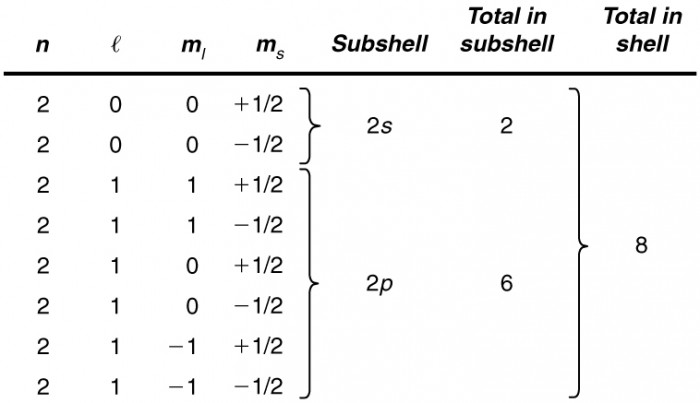Figure 4

#### Discussion

It is laborious to make a table like this every time we want to know how many electrons can be in a shell or subshell. There exist general rules that are easy to apply, as we shall now see.

The number of electrons that can be in a subshell depends entirely on the value of l. Once l is known, there are a fixed number of values of ml, each of which can have two values for ms First, since ml goes from l to l in steps of 1, there are 2l + 1 possibilities. This number is multiplied by 2, since each electron can be spin up or spin down. Thus the maximum number of electrons that can be in a subshell is 2(2l + 1).

For example, the 2s subshell in Example 1 has a maximum of 2 electrons in it, since 2(2+ 1) = 2(0 + 1) = 2 for this subshell. Similarly, the 2p subshell has a maximum of 6 electrons, since 2(2+ 1) = 2(2 + 1) = 6. For a shell, the maximum number is the sum of what can fit in the subshells. Some algebra shows that the maximum number of electrons that can be in a shell is 2n2.

For example, for the first shell = 1, and so 2n2 = 2. We have already seen that only two electrons can be in the = 1 shell. Similarly, for the second shell, = 2, and so 2n2 = 8. As found in Example 1, the total number of electrons in the = 2 shell is 8.

### Example 2. Subshells and Totals for $n=3\\$

How many subshells are in the = 3 shell? Identify each subshell, calculate the maximum number of electrons that will fit into each, and verify that the total is 2n2.

#### Strategy

Subshells are determined by the value of l; thus, we first determine which values of l are allowed, and then we apply the equation “maximum number of electrons that can be in a subshell = 2(2+ 1)” to find the number of electrons in each subshell.

#### Solution

Since n=3, we know that l can be 0, 1, or 2; thus, there are three possible subshells. In standard notation, they are labeled the 3s, 3p, and 3d subshells. We have already seen that 2 electrons can be in an s state, and 6 in a p state, but let us use the equation “maximum number of electrons that can be in a subshell = 2(2+ 1)” to calculate the maximum number in each:

3s has l = 0; thus 2(2l + 1) = 2(0 + 1) = 2

3p has l = 1; thus, 2(2l + 1) = 2(2 + 1) = 6

3d has l = 2; thus 2(2l + 1) = 2(4 + 1) = 10

Total = 18

(in the n = 3 shell)

The equation “maximum number of electrons that can be in a shell = 2n2” gives the maximum number in the = 3 shell to be:

Maximum number of electrons = 2n2 = 2(3)2 = 2(9) = 18.

#### Discussion

The total number of electrons in the three possible subshells is thus the same as the formula 2n2. In standard (spectroscopic) notation, a filled = 3 shell is denoted as 3s23p63d10. Shells do not fill in a simple manner. Before the = 3 shell is completely filled, for example, we begin to find electrons in the = 4 shell.

## Shell Filling and the Periodic Table

Table 2 shows electron configurations for the first 20 elements in the periodic table, starting with hydrogen and its single electron and ending with calcium. The Pauli exclusion principle determines the maximum number of electrons allowed in each shell and subshell. But the order in which the shells and subshells are filled is complicated because of the large numbers of interactions between electrons.

Table 2. Electron Configurations of Elements Hydrogen Through Calcium
Element Number of electrons (Z) Ground state configuration
H 1 1s1
He 2 1s2
Li 3 1s2 2s1
Be 4 2s2
B 5 2s2 2p1
C 6 2s2 2p2
N 7 2s2 2p3
O 8 2s2 2p4
F 9 2s2 2p5
Ne 10 2s2 2p6
Na 11 2s2 2p6 3s1
Mg 12 3s2
Al 13 3s2 3p1
Si 14 3s2 3p2
P 15 3s2 3p3
S 16 3s2 3p4
Cl 17 3s2 3p5
Ar 18 3s2 3p6
K 19 3s2 3p6 4s1
Ca 20 4s2

Examining the above table, you can see that as the number of electrons in an atom increases from 1 in hydrogen to 2 in helium and so on, the lowest-energy shell gets filled first—that is, the = 1 shell fills first, and then the = 2 shell begins to fill. Within a shell, the subshells fill starting with the lowest l, or with the s subshell, then the p, and so on, usually until all subshells are filled. The first exception to this occurs for potassium, where the 4s subshell begins to fill before any electrons go into the 3d subshell. The next exception is not shown in Table 2; it occurs for rubidium, where the 5s subshell starts to fill before the 4d subshell. The reason for these exceptions is that = 0 electrons have probability clouds that penetrate closer to the nucleus and, thus, are more tightly bound (lower in energy).

Figure 5 shows the periodic table of the elements, through element 118. Of special interest are elements in the main groups, namely, those in the columns numbered 1, 2, 13, 14, 15, 16, 17, and 18.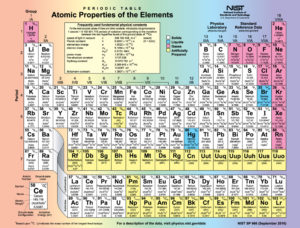Figure 5. Periodic table of the elements (credit: National Institute of Standards and Technology, U.S. Department of Commerce)

The number of electrons in the outermost subshell determines the atom’s chemical properties, since it is these electrons that are farthest from the nucleus and thus interact most with other atoms. If the outermost subshell can accept or give up an electron easily, then the atom will be highly reactive chemically. Each group in the periodic table is characterized by its outermost electron configuration. Perhaps the most familiar is Group 18 (Group VIII), the noble gases (helium, neon, argon, etc.). These gases are all characterized by a filled outer subshell that is particularly stable. This means that they have large ionization energies and do not readily give up an electron. Furthermore, if they were to accept an extra electron, it would be in a significantly higher level and thus loosely bound. Chemical reactions often involve sharing electrons. Noble gases can be forced into unstable chemical compounds only under high pressure and temperature.

Group 17 (Group VII) contains the halogens, such as fluorine, chlorine, iodine and bromine, each of which has one less electron than a neighboring noble gas. Each halogen has 5 p electrons (a p5 configuration), while the p subshell can hold 6 electrons. This means the halogens have one vacancy in their outermost subshell. They thus readily accept an extra electron (it becomes tightly bound, closing the shell as in noble gases) and are highly reactive chemically. The halogens are also likely to form singly negative ions, such as C1, fitting an extra electron into the vacancy in the outer subshell. In contrast, alkali metals, such as sodium and potassium, all have a single s electron in their outermost subshell (an s1 configuration) and are members of Group 1 (Group I). These elements easily give up their extra electron and are thus highly reactive chemically. As you might expect, they also tend to form singly positive ions, such as Na+, by losing their loosely bound outermost electron. They are metals (conductors), because the loosely bound outer electron can move freely.

Of course, other groups are also of interest. Carbon, silicon, and germanium, for example, have similar chemistries and are in Group 4 (Group IV). Carbon, in particular, is extraordinary in its ability to form many types of bonds and to be part of long chains, such as inorganic molecules. The large group of what are called transitional elements is characterized by the filling of the d subshells and crossing of energy levels. Heavier groups, such as the lanthanide series, are more complex—their shells do not fill in simple order. But the groups recognized by chemists such as Mendeleev have an explanation in the substructure of atoms.

## PhET Explorations: Build an Atom

Build an atom out of protons, neutrons, and electrons, and see how the element, charge, and mass change. Then play a game to test your ideas!

## Section Summary

• The state of a system is completely described by a complete set of quantum numbers. This set is written as (n, l, ml, ms).
• The Pauli exclusion principle says that no two electrons can have the same set of quantum numbers; that is, no two electrons can be in the same state.
• This exclusion limits the number of electrons in atomic shells and subshells. Each value of n corresponds to a shell, and each value of l corresponds to a subshell.
• The maximum number of electrons that can be in a subshell is 2(2l + 1).
• The maximum number of electrons that can be in a shell is 2n2.

### Conceptual Questions

1. Identify the shell, subshell, and number of electrons for the following: (a) 2p3. (b) 4d9. (c) 3s1. (d) 5g16.
2. Which of the following are not allowed? State which rule is violated for any that are not allowed. (a) 1p3 (b) 2p8 (c) 3g11 (d) 4f2

### Problems & Exercises

1. (a) How many electrons can be in the n=4 shell? (b) What are its subshells, and how many electrons can be in each?
2. (a) What is the minimum value of 1 for a subshell that has 11 electrons in it? (b) If this subshell is in the n = 5 shell, what is the spectroscopic notation for this atom?
3. (a) If one subshell of an atom has 9 electrons in it, what is the minimum value of l? (b) What is the spectroscopic notation for this atom, if this subshell is part of the n = 3 shell?
4. (a) List all possible sets of quantum numbers (n, l, ml, ms) for the n = 3 shell, and determine the number of electrons that can be in the shell and each of its subshells. (b) Show that the number of electrons in the shell equals 2n2 and that the number in each subshell is 2(2l + 1).
5. Which of the following spectroscopic notations are not allowed? (a) 5s1 (b) 1d1 (c) 4s3 (d) 3p7 (e) 5g15. State which rule is violated for each that is not allowed.
6. Which of the following spectroscopic notations are allowed (that is, which violate none of the rules regarding values of quantum numbers)? (a) 1s1 (b) 1d3 (c) 4s2 (d) 3p7 (e) 6h20
7. (a) Using the Pauli exclusion principle and the rules relating the allowed values of the quantum numbers (n, l, ml, ms), prove that the maximum number of electrons in a subshell is 2n2. (b) In a similar manner, prove that the maximum number of electrons in a shell is 2n2.
8. Integrated Concepts. Estimate the density of a nucleus by calculating the density of a proton, taking it to be a sphere 1.2 fm in diameter. Compare your result with the value estimated in this chapter.
9. Integrated Concepts. The electric and magnetic forces on an electron in the CRT in Figure 6 are supposed to be in opposite directions. Verify this by determining the direction of each force for the situation shown. Explain how you obtain the directions (that is, identify the rules used).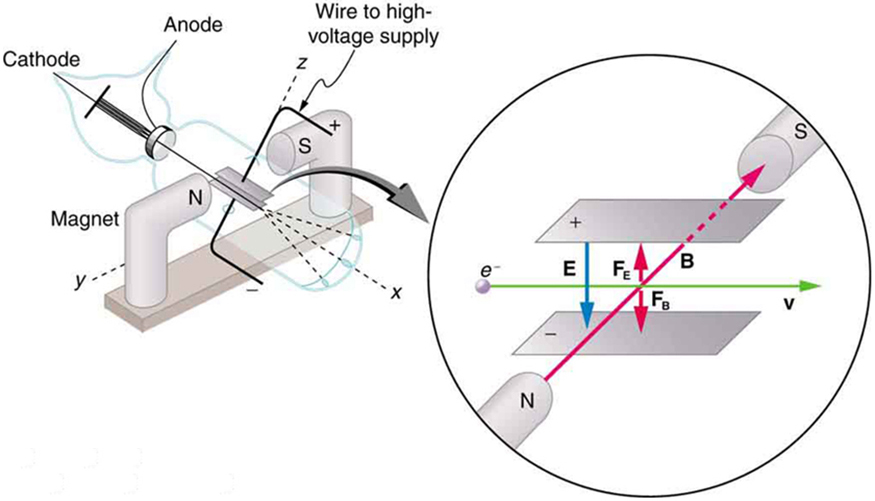Figure 6. This schematic shows the electron beam in a CRT passing through crossed electric and magnetic fields and causing phosphor to glow when striking the end of the tube.

10. (a) What is the distance between the slits of a diffraction grating that produces a first-order maximum for the first Balmer line at an angle of 20.0º? (b) At what angle will the fourth line of the Balmer series appear in first order? (c) At what angle will the second-order maximum be for the first line?
11. Integrated Concepts. A galaxy moving away from the earth has a speed of 0.0100c. What wavelength do we observe for an ni = 7 to nf = 2 transition for hydrogen in that galaxy?
12. Integrated Concepts. Calculate the velocity of a star moving relative to the earth if you observe a wavelength of 91.0 nm for ionized hydrogen capturing an electron directly into the lowest orbital (that is, a ni = ∞ to nf = 1, or a Lyman series transition).
13. Integrated Concepts. In a Millikan oil-drop experiment using a setup like that in Figure 7, a 500-V potential difference is applied to plates separated by 2.50 cm. (a) What is the mass of an oil drop having two extra electrons that is suspended motionless by the field between the plates? (b) What is the diameter of the drop, assuming it is a sphere with the density of olive oil?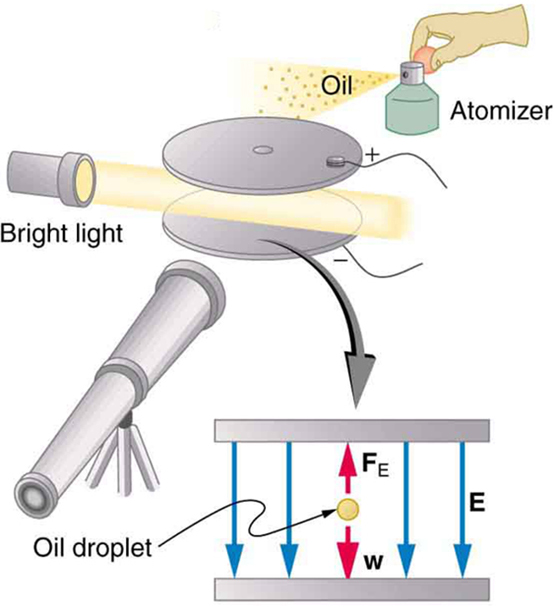Figure 7. The Millikan oil drop experiment produced the first accurate direct measurement of the charge on electrons, one of the most fundamental constants in nature. Fine drops of oil become charged when sprayed. Their movement is observed between metal plates with a potential applied to oppose the gravitational force. The balance of gravitational and electric forces allows the calculation of the charge on a drop. The charge is found to be quantized in units of −1.6 × 10−19 C, thus determining directly the charge of the excess and missing electrons on the oil drops.

14. Integrated Concepts. What double-slit separation would produce a first-order maximum at 3.00º for 25.0-keV x rays? The small answer indicates that the wave character of x rays is best determined by having them interact with very small objects such as atoms and molecules.
15. Integrated Concepts. In a laboratory experiment designed to duplicate Thomson’s determination of $\frac{{q}_{e}}{{m}_{e}}\\$, a beam of electrons having a velocity of 6.00 × 107 m/s enters a 5.00 × 10−3 T magnetic field. The beam moves perpendicular to the field in a path having a 6.80-cm radius of curvature. Determine $\frac{{q}_{e}}{{m}_{e}}\\$ from these observations, and compare the result with the known value.
16. Integrated Concepts. Find the value of l, the orbital angular momentum quantum number, for the moon around the earth. The extremely large value obtained implies that it is impossible to tell the difference between adjacent quantized orbits for macroscopic objects.
17. Integrated Concepts. Particles called muons exist in cosmic rays and can be created in particle accelerators. Muons are very similar to electrons, having the same charge and spin, but they have a mass 207 times greater. When muons are captured by an atom, they orbit just like an electron but with a smaller radius, since the mass in ${a}_{\text{B}}=\frac{{h}^{2}}{{4\pi }^{2}{m}_{e}{\text{kq}}_{e}^{2}}=\text{0.529}\times {\text{10}}^{-\text{10}}\text{m}\\$ is 207 me. (a) Calculate the radius of the n = 1 orbit for a muon in a uranium ion (= 92). (b) Compare this with the 7.5-fm radius of a uranium nucleus. Note that since the muon orbits inside the electron, it falls into a hydrogen-like orbit. Since your answer is less than the radius of the nucleus, you can see that the photons emitted as the muon falls into its lowest orbit can give information about the nucleus.
18. Integrated Concepts. Calculate the minimum amount of energy in joules needed to create a population inversion in a helium-neon laser containing 1.00 × 10−4 moles of neon.
19. Integrated Concepts. A carbon dioxide laser used in surgery emits infrared radiation with a wavelength of 10.6 µm. In 1.00 ms, this laser raised the temperature of 1.00 cm3 of flesh to 100ºC and evaporated it. (a) How many photons were required? You may assume flesh has the same heat of vaporization as water. (b) What was the minimum power output during the flash?
20. Integrated Concepts. Suppose an MRI scanner uses 100-MHz radio waves. (a) Calculate the photon energy. (b) How does this compare to typical molecular binding energies?
21. Integrated Concepts. (a) An excimer laser used for vision correction emits 193-nm UV. Calculate the photon energy in eV. (b) These photons are used to evaporate corneal tissue, which is very similar to water in its properties. Calculate the amount of energy needed per molecule of water to make the phase change from liquid to gas. That is, divide the heat of vaporization in kJ/kg by the number of water molecules in a kilogram. (c) Convert this to eV and compare to the photon energy. Discuss the implications.
22. Integrated Concepts. A neighboring galaxy rotates on its axis so that stars on one side move toward us as fast as 200 km/s, while those on the other side move away as fast as 200 km/s. This causes the EM radiation we receive to be Doppler shifted by velocities over the entire range of ±200 km/s. What range of wavelengths will we observe for the 656.0-nm line in the Balmer series of hydrogen emitted by stars in this galaxy. (This is called line broadening.)
23. Integrated Concepts. A pulsar is a rapidly spinning remnant of a supernova. It rotates on its axis, sweeping hydrogen along with it so that hydrogen on one side moves toward us as fast as 50.0 km/s, while that on the other side moves away as fast as 50.0 km/s. This means that the EM radiation we receive will be Doppler shifted over a range of ±50.0 km/s. What range of wavelengths will we observe for the 91.20-nm line in the Lyman series of hydrogen? (Such line broadening is observed and actually provides part of the evidence for rapid rotation.)
24. Integrated Concepts. Prove that the velocity of charged particles moving along a straight path through perpendicular electric and magnetic fields is $v=\frac{E}{B}\\$. Thus crossed electric and magnetic fields can be used as a velocity selector independent of the charge and mass of the particle involved.
25. Unreasonable Results. (a) What voltage must be applied to an X-ray tube to obtain 0.0100-fm-wavelength X-rays for use in exploring the details of nuclei? (b) What is unreasonable about this result? (c) Which assumptions are unreasonable or inconsistent?
26. Unreasonable Results. A student in a physics laboratory observes a hydrogen spectrum with a diffraction grating for the purpose of measuring the wavelengths of the emitted radiation. In the spectrum, she observes a yellow line and finds its wavelength to be 589 nm. (a) Assuming this is part of the Balmer series, determine ni, the principal quantum number of the initial state. (b) What is unreasonable about this result? (c) Which assumptions are unreasonable or inconsistent?
27. Construct Your Own Problem. The solar corona is so hot that most atoms in it are ionized. Consider a hydrogen-like atom in the corona that has only a single electron. Construct a problem in which you calculate selected spectral energies and wavelengths of the Lyman, Balmer, or other series of this atom that could be used to identify its presence in a very hot gas. You will need to choose the atomic number of the atom, identify the element, and choose which spectral lines to consider.
28. Construct Your Own Problem. Consider the Doppler-shifted hydrogen spectrum received from a rapidly receding galaxy. Construct a problem in which you calculate the energies of selected spectral lines in the Balmer series and examine whether they can be described with a formula like that in the equation $\frac{1}{\lambda }=R\left(\frac{1}{{n}_{\text{f}}^{2}}-\frac{1}{{n}_{\text{i}}^{2}}\right)\\$, but with a different constant R.

## Glossary

atomic number: the number of protons in the nucleus of an atom

Pauli exclusion principle: a principle that states that no two electrons can have the same set of quantum numbers; that is, no two electrons can be in the same state

shell: a probability cloud for electrons that has a single principal quantum number

subshell: the probability cloud for electrons that has a single angular momentum quantum number l

### Selected Solutions to Problems & Exercises

1. (a) 32. (b) 2 in s,6 in p,10 in d, and 14 in f, for a total of 32.

3. (a) 2; (b) 3d9

5. (b) ≥ l is violated; (c) cannot have 3 electrons in s subshell since 3 > (2l + 1)=2; (d) cannot have 7 electrons in p subshell since 7 > (2l + 1) = 2(2 + 1) = 6

7.  (a) The number of different values of ml is ±l, (l − 1), . . . , 0 for each > 0 and one for $l=0\Rightarrow \left(2l+1\right)\\$. Also an overall factor of 2 since each ml can have ms equal to either +1/2 or $-1/2\Rightarrow 2\left(2l+1\right)\\$.

(b) for each value of l, you get $2\left(2l+1\right)=\text{0, 1, 2, …,}\left(n\text{-1}\right)\Rightarrow$

$\begin{array}{l}2\left\{\left[\left(2\right)\left(0\right)+1\right]+\left[\left(2\right)\left(1\right)+1\right]+\dots+\left[\left(2\right)\left(n - 1\right)+1\right]\right\}=\\\underset{n\text{ terms}}{\underbrace{2\left[1+3+\dots+\left(2n-3\right)+\left(2n-1\right)\right]}}\end{array}\\$

to see that the expression in the box is = n2, imagine taking (n − 1) from the last term and adding it to first term:

$\begin{array}{ll}=&2\left[1+\left(n-1\right)+3+\dots+\left(2n-3\right)+\left(2n-1\right)-\left(n-1\right)\right]\\=&2\left[n+3+\dots+\left(2n-3\right)+n\right]\end{array}\\$

Now take (n − 3) from penultimate term and add to the second term $2\underset{n\text{ terms}}{\underbrace{\left[n+n+\dots+n+n\right]}}={2n}^{2}\\$.

9. The electric force on the electron is up (toward the positively charged plate). The magnetic force is down (by the RHR).

11. 401 nm

13. (a) 6.54 × 10−16 kg; (b) 5.54 × 10−7 m

15. 1.76 × 1011 C/kg, which agrees with the known value of 1.759 × 1011 C/kg to within the precision of the measurement

17. (a) 2.78 fm; (b) 0.37 of the nuclear radius.

19. (a) 1.34 × 1023; (b) 2.52 MW

21. (a) 6.42 eV; (b) 7.27 × 10−20 J/molecule; (c) 0.454 eV, 14.1 times less than a single UV photon. Therefore, each photon will evaporate approximately 14 molecules of tissue. This gives the surgeon a rather precise method of removing corneal tissue from the surface of the eye.

23. 91.18 nm to 91.22 nm

25. (a) 1.24 × 1011 V; (b) The voltage is extremely large compared with any practical value; (c) The assumption of such a short wavelength by this method is unreasonable.

1. It is unusual to deal with subshells having l greater than 6, but when encountered, they continue to be labeled in alphabetical order.